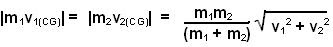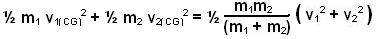## Wednesday, June 4, 2008

### Irodov Problem 1.174The velocity vectors of masses m1 and m2 are given by (v1 i + 0 j) and (o i + v2 j) respectively. The velocity of the center of mass is then given by,The velocities of the particles relative to a frame fixed to the CG are given by,Thus, momenta of either particles as seen from the center of mass will beThe kinetic energy of the entire system as seen from CG will be given by,